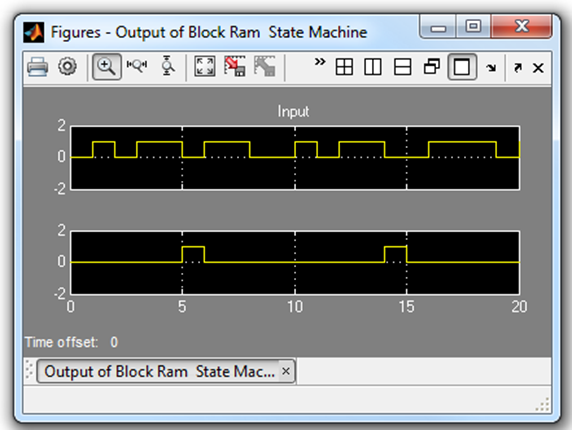# Step 1: Modeling Control with M-Code - 2020.2 English

Document ID
UG1498
Release Date
2021-01-22
Version
2020.2 English
In this step you will be creating a simple Finite State Machine (FSM) using the MCode block to detect a sequence of binary values 1011. The FSM needs to be able to detect multiple transmissions as well, such as 10111011.

## Objectives

After completing this lab, you will be able to create a Finite State Machine using the MCode block in System Generator.

## Procedure

In this step you will create the control logic for a Finite State Machine using M-code. You will then simulate the final design to confirm the correct operation.

1. Launch System Generator and change the working directory to: C:\SysGen_Tutorial\Lab2\M_code
2. Open the file Lab2_1.slx.

You see the following incomplete diagram.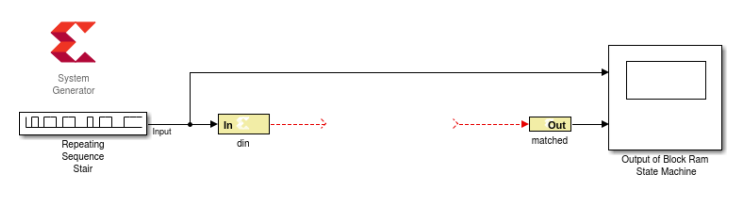3. Add an MCode block from the Xilinx® Blockset/Index library. Before wiring up the block, you need to edit the MATLAB® function to create the correct ports and function name.
4. Double-click the MCode block and click Edit M-File, as shown in the following figure.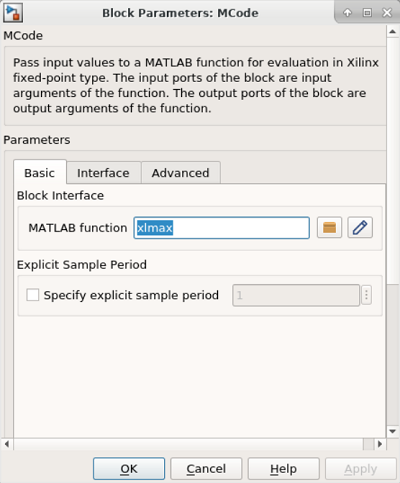The following figure shows the default M-code in the MATLAB text editor.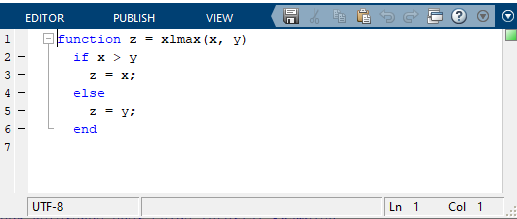5. Edit the default MATLAB function to include the function name `state_machine` and the input `din` and output `matched`.
6. You can now delete the sample M-code.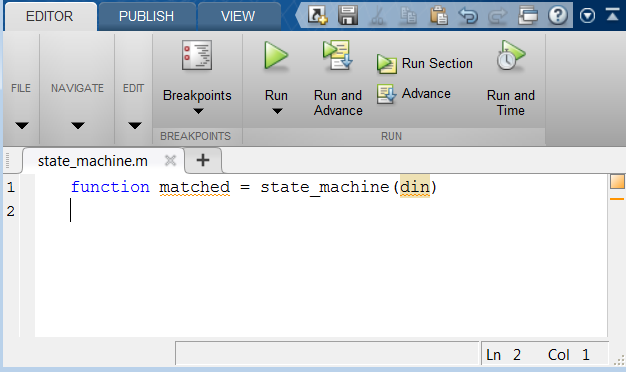7. After you make the edits, use Save As to save the MATLAB file as state_machine.m to the Lab2 folder.
1. In the MCode Properties Editor, use the Browse button to ensure that the MCode block is referencing the local M-code file (state_machine.m).
8. In the MCode Properties Editor, click OK.

You will see the MCode block assume the new ports and function name.

9. Now connect the MCode block to the diagram as shown in the following figure: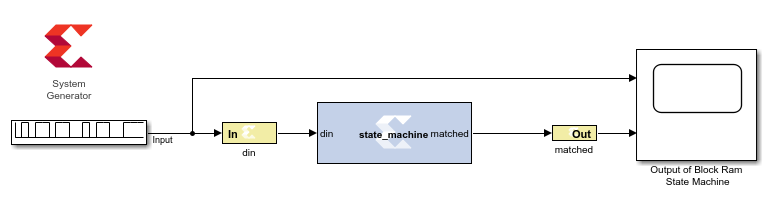You are now ready to start coding the state machine. The bubble diagram for this state machine is shown in the following figure. This FSM has five states and is capable of detecting two sequences in succession.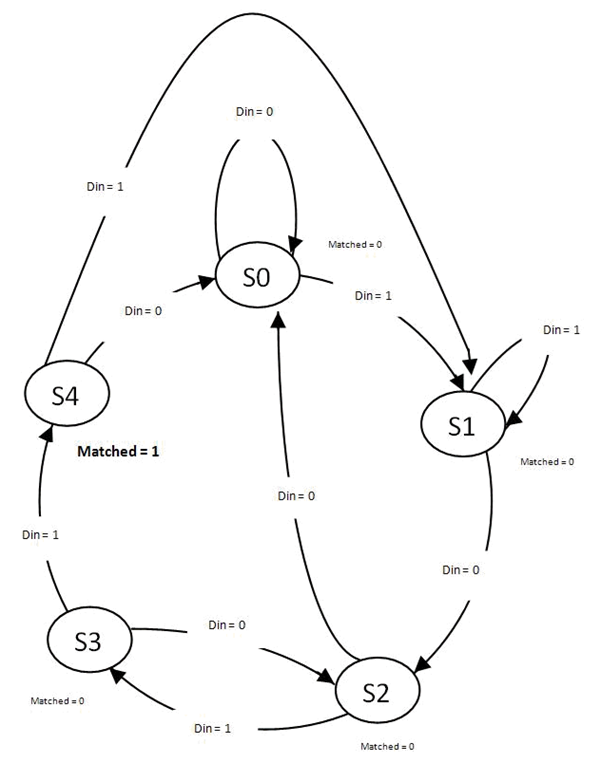10. Edit the M-code file, state_machine.m, and define the state variable using the Xilinx `xl_state` data type as shown in the following. This requires that you declare a variable as a persistent variable. The `xl_state` function requires two arguments: the initial condition and a fixed-point declaration.

Because you need to count up to 4, you need 3 bits.

```persistent state, state = xl_state(0,{xlUnsigned, 3, 0});```

11. Use a switch-case statement to define the FSM states shown. A small sample is provided, shown as follows, to get you started.
Note: You need an otherwise statement as your last case.
``````switch state
case 0
if din == 1
state = 1
else
state = 0
end
matched = 0;``````
12. Save the M-code file and run the simulation. The waveform should look like the following figure.

You should notice two detections of the sequence.